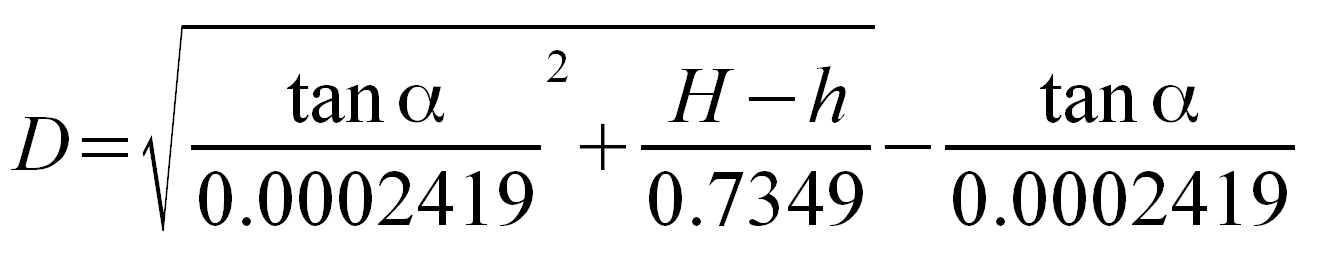Table 15: Distance by vertical angle measured between
sea horizon and top of object beyond sea horizon

 Angle: °  ' Height of object: feet yards meters statute miles nautical miles Height of eye: inches feet yards meters statute miles nautical miles in feet yards meters statute miles nautical milesα = corrected vertical angle (degrees) H = height of object above sea level (feet) h = height of eye above sea level (feet) D = distance (nm)